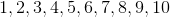# Common Core: Kindergarten Math : Counting & Cardinality

## Example Questions

### Example Question #105 : Comparing Two Numbers Between 1 And 10: Ccss.Math.Content.K.Cc.C.7

Fill in the blank.__________is less than

is equal to

is greater than

is greater than

Explanation:is greater thanbecausecomes afterwhen we are counting, which means it is greater.### Example Question #106 : Comparing Two Numbers Between 1 And 10: Ccss.Math.Content.K.Cc.C.7

Fill in the blank.__________is less than

is greater than

is equal to

is greater than

Explanation:is greater thanbecausecomes afterwhen we are counting, which means it is greater.### Example Question #107 : Comparing Two Numbers Between 1 And 10: Ccss.Math.Content.K.Cc.C.7

Fill in the blank.__________is less than

is equal to

is greater than

is greater than

Explanation:is greater thanbecausecomes afterwhen we are counting, which means it is greater.### Example Question #108 : Comparing Two Numbers Between 1 And 10: Ccss.Math.Content.K.Cc.C.7

Fill in the blank.__________is greater than

is equal to

is less than

is greater than

Explanation:is greater thanbecausecomes afterwhen we are counting, which means it is greater.### Example Question #109 : Comparing Two Numbers Between 1 And 10: Ccss.Math.Content.K.Cc.C.7

Fill in the blank.__________is equal to

is less than

is greater than

is greater than

Explanation:is greater thanbecausecomes afterwhen we are counting, which means it is greater.### Example Question #110 : Comparing Two Numbers Between 1 And 10: Ccss.Math.Content.K.Cc.C.7

Fill in the blank.__________is greater than

is equal to

is less than

is greater than

Explanation:is greater thanbecausecomes afterwhen we are counting, which means it is greater.### Example Question #111 : Comparing Two Numbers Between 1 And 10: Ccss.Math.Content.K.Cc.C.7

Fill in the blank.__________is less than

is equal to

is greater than

is equal to

Explanation:andare the same number which means they are equal.

### Example Question #112 : Comparing Two Numbers Between 1 And 10: Ccss.Math.Content.K.Cc.C.7

Fill in the blank.__________is less than

is greater than

is equal to

is less than

Explanation:is less thanbecausecomes beforewhen we are counting, which means it is less.### Example Question #113 : Comparing Two Numbers Between 1 And 10: Ccss.Math.Content.K.Cc.C.7

Fill in the blank.__________is greater than

is less than

is equal to

is greater than

Explanation:is greater thanbecausecomes afterwhen we are counting, which means it is greater.### Example Question #114 : Comparing Two Numbers Between 1 And 10: Ccss.Math.Content.K.Cc.C.7

Fill in the blank.__________is less than

is equal to

is greater thanis greater thanbecausecomes afterwhen we are counting, which means it is greater.